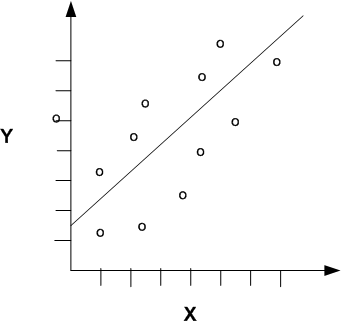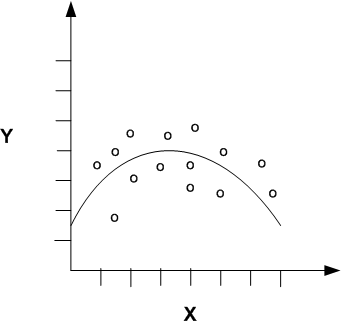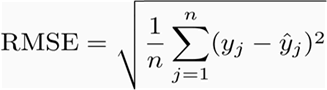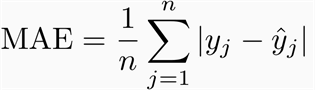# 4 Regression

This chapter describes regression, the supervised mining function for predicting a numerical target.

"Supervised Data Mining"

This chapter includes the following topics:

Regression is a data mining function that predicts a number. Age, weight, distance, temperature, income, or sales could all be predicted using regression techniques. For example, a regression model could be used to predict children's height, given their age, weight, and other factors.

A regression task begins with a data set in which the target values are known. For example, a regression model that predicts children's height could be developed based on observed data for many children over a period of time. The data might track age, height, weight, developmental milestones, family history, and so on. Height would be the target, the other attributes would be the predictors, and the data for each child would constitute a case.

In the model build (training) process, a regression algorithm estimates the value of the target as a function of the predictors for each case in the build data. These relationships between predictors and target are summarized in a model, which can then be applied to a different data set in which the target values are unknown.

Regression models are tested by computing various statistics that measure the difference between the predicted values and the expected values. See "Testing a Regression Model".

### Common Applications of Regression

Regression modeling has many applications in trend analysis, business planning, marketing, financial forecasting, time series prediction, biomedical and drug response modeling, and environmental modeling.

### How Does Regression Work?

You do not need to understand the mathematics used in regression analysis to develop quality regression models for data mining. However, it is helpful to understand a few basic concepts.

The goal of regression analysis is to determine the values of parameters for a function that cause the function to best fit a set of data observations that you provide. The following equation expresses these relationships in symbols. It shows that regression is the process of estimating the value of a continuous target (y) as a function (F) of one or more predictors (x1 , x2 , ..., xn), a set of parameters (θ1 , θ2 , ..., θn), and a measure of error (e).

```y = F(x,θ)  + e
```

The process of training a regression model involves finding the best parameter values for the function that minimize a measure of the error, for example, the sum of squared errors.

There are different families of regression functions and different ways of measuring the error.

#### Linear Regression

The simplest form of regression to visualize is linear regression with a single predictor. A linear regression technique can be used if the relationship between x and y can be approximated with a straight line, as shown in Figure 4-1.

Figure 4-1 Linear Relationship Between x and yDescription of "Figure 4-1 Linear Relationship Between x and y"

In a linear regression scenario with a single predictor (y = θ2x + θ1), the regression parameters (also called coefficients) are:

The slope of the line (θ2) — the angle between a data point and the regression line
and
The y intercept (θ1) — the point where x crosses the y axis (x = 0)

#### Nonlinear Regression

Often the relationship between x and y cannot be approximated with a straight line. In this case, a nonlinear regression technique may be used. Alternatively, the data could be preprocessed to make the relationship linear.

In Figure 4-2, x and y have a nonlinear relationship. Oracle Data Mining supports nonlinear regression via the gaussian kernel of SVM. (See "Kernel-Based Learning".)

Figure 4-2 Nonlinear Relationship Between x and yDescription of "Figure 4-2 Nonlinear Relationship Between x and y"

#### Multivariate Regression

Multivariate regression refers to regression with multiple predictors (x1 , x2 , ..., xn). For purposes of illustration, Figure 4-1 and Figure 4-2 show regression with a single predictor. Multivariate regression is also referred to as multiple regression.

## Regression Algorithms

Oracle Data Mining provides the following algorithms for regression:

• Generalized Linear Models

Generalized Linear Models (GLM) is a popular statistical technique for linear modeling. Oracle Data Mining implements GLM for regression and classification. See Chapter 12, "Generalized Linear Models"

• Support Vector Machines

Support Vector Machines (SVM) is a powerful, state-of-the-art algorithm for linear and nonlinear regression. Oracle Data Mining implements SVM for regression and other mining functions. See Chapter 18, "Support Vector Machines"

Note:

Both GLM and SVM, as implemented by Oracle Data Mining, are particularly suited for mining data that includes many predictors (wide data).

## Testing a Regression Model

The Root Mean Squared Error and the Mean Absolute Error are statistics for evaluating the overall quality of a regression model. Different statistics may also be available depending on the regression methods used by the algorithm.

### Root Mean Squared Error

The Root Mean Squared Error (RMSE) is the square root of the average squared distance of a data point from the fitted line. Figure 4-3 shows the formula for the RMSE.

Figure 4-3 Root Mean Squared ErrorDescription of "Figure 4-3 Root Mean Squared Error"

This SQL expression calculates the RMSE.

```SQRT(AVG((predicted_value - actual_value) * (predicted_value - actual_value)))
```

### Mean Absolute Error

The Mean Absolute Error (MAE) is the average of the absolute value of the residuals. The MAE is very similar to the RMSE but is less sensitive to large errors. Figure 4-4 shows the formula for the MAE.

Figure 4-4 Mean Absolute ErrorDescription of "Figure 4-4 Mean Absolute Error"

This SQL expression calculates the MAE.

```AVG(ABS(predicted_value - actual_value))
```